• set有两个相似的内置函数：difference()返回多个集合的差集symmetric_difference()返回两个集合中不重复的元素集合什么意思呢？被困惑了一下，各种try, try, try… 终于，明白了。看例子吧！# 定义三个seta = set('...

set有两个相似的内置函数：

difference()返回多个集合的差集symmetric_difference()返回两个集合中不重复的元素集合

什么意思呢？被困惑了一下，各种try, try, try… 终于，明白了。

看例子吧！

# 定义三个set

a = set('abcd')

b = set('cdef')

c = set('bcde')

# difference() vs. symmetric_difference()

>>>a.difference(b)

{'a', 'b'}

>>>b.difference(a)

{'e', 'f'}

>>>a.symmetric_difference(b)

{'a', 'e', 'f', 'b'}

>>>b.symmetric_difference(a)

{'a', 'e', 'f', 'b'}

相信看过例子，大家就都明白了。

总结一下

difference()返回的新set的元素：包含在a中，并且不包含在集合b中 。 所以a.difference(b) 和b.difference(a)的结果是不一样的。 a.difference(b) 等效于 a - b b.difference(a) 等效于 b - a  symmetric_difference(), 此时再体会一下symmetric的含义就清楚了，对称的，所以返回的新set的元素：包含在a中，并且不包含在集合b中 + 包含在b中，并且不包含在集合a中。 这么解释反而复杂了，其实简单来说就是去重。

补充一点 difference()方法的参数可以是一个或多个set。 下面是带多个参数的例子：

# c = set('bcde')

>>>a.difference(b)

{'a', 'b'}

>>>a.difference(b, c)

{'a'}

返回的set中的元素： 包含在a中，并且不包含在集合b和c中

展开全文• 论文简介 人脸反欺骗（Face anti-spoofing，FAS）中的...因此本文提出一种新的卷积操作——中心差分卷积（Central Difference Convolution，CDC），并基于这种卷积操作提出了一种新的frame-level人脸反欺骗结构。该方法

Searching Central Difference Convolutional Networks for Face Anti-Spoofing

论文简介

人脸反欺骗（Face Anti-SpoofingFAS）中的大多数最先进的方法往往存在以下缺点：
1)都是通过设计专门的网络结构和堆叠卷积操作实现的，这种方法难以描述图像的细粒度信息，并且这样的方法容易受到环境变化的影响（如照明不同）
2)采用多帧输入的方式，实时性方面欠缺，难以部署到实时响应的环境当中

因此本文提出一种新的卷积操作——中心差分卷积Central Difference ConvolutionCDC），并基于这种卷积操作提出了一种新的frame-level人脸反欺骗结构。该方法能够通过汇总强度和梯度信息来捕获本质的细节信息（模式）。使用CDC构建的网络称为CDCN，与使用传统卷积操作构建的网络相比，CDCN能够提供更强大的建模能力。并且在经过专门的CDC搜索空间上，神经结构搜索NAS）可用于发现更强大的网络结构（CDCN++），这种网络将其与多尺度注意力模块MAFM）组装在一起可以进一步提高性能。实验结果表明：
1)本文的方法不仅在内部测试表现出优异的性能（OULU-NPU协议1取得了 0.2 % A C E R 0.2\% ACER
2)在跨数据集测试中也具有很好的泛化性（尤其是从CASIA-MFSD到Replay-Attack的 6.5 ％ H T E R 6.5％HTER

论文研读

Introduction

人脸识别已在许多交互式人工智能系统中广泛使用来提供便捷的操作体验。但是，存在的欺骗人脸限制了其可靠的部署。仅将打印的图像或视频呈现给生物识别传感器就可能使人脸识别系统做出错误的判断。经典的演示攻击案例包括视频重放攻击、打印攻击和3D面罩攻击。为了保证人脸识别的可靠性，人脸反欺骗方法对于检测这些攻击显得尤为重要

多数近期的使用深度学习的FAS方法通常基于图像分类任务的backbone，例如VGG、ResNet、以及DenseNet。这些网络通常使用二分类交叉熵损失函数进行监督训练，这很容易导致网络学到一些分散的信息（例如屏幕边框等）而难以归纳出本质的特征为了解决这个问题，已经开发了利用伪深度图像作为辅助监督信号的FAS网路结构但是，这些网络都是由专业人员精心设计的，对于FAS任务而言，这可能并不是最佳选择。因此，应考虑使用辅助的深度图监督来自动的发现最合适FAS任务的网络结构

基于以上讨论，我们提出了一种新颖的卷积算子，称为“中心差分卷积”（CDC），它擅长描述细粒度的不变信息。如下图所示，在各种环境中，CDC比传统的卷积操作更有可能提取出固有的欺骗模式（例如，晶格伪影）。此外，在专门设计的**CDC搜索空间**上，神经结构搜索NAS）用于发现使用深度图监督的人脸反欺骗任务的出色frame-level网络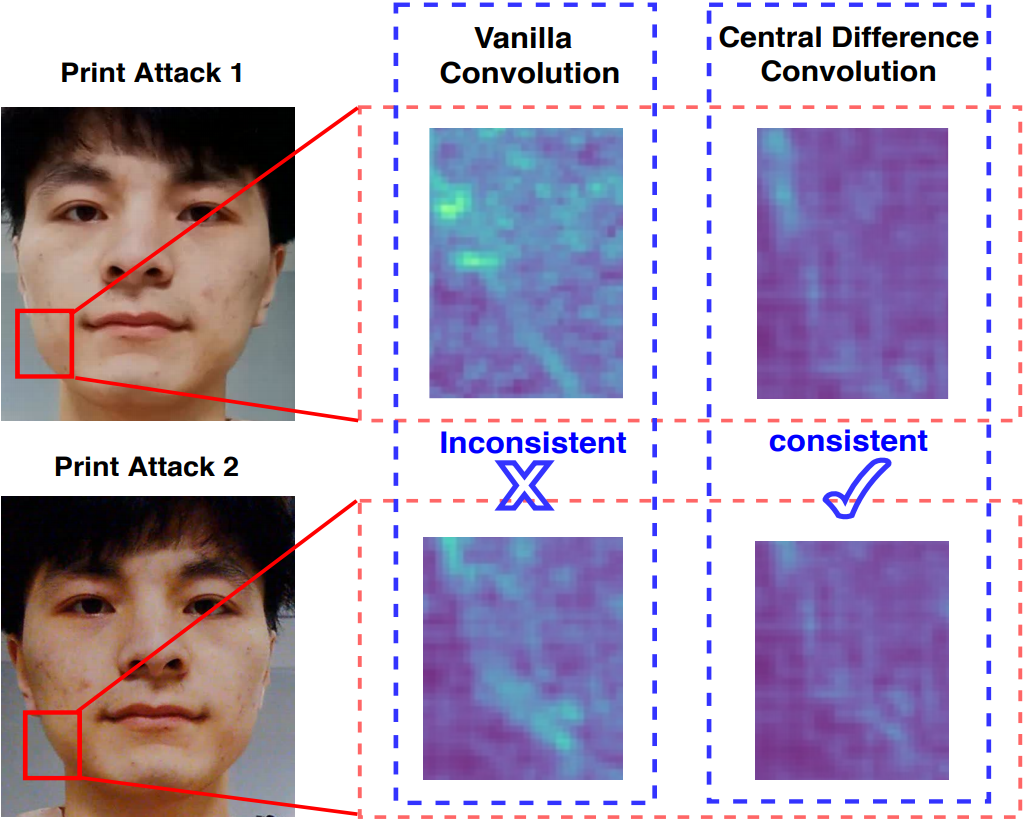Feature response of vanilla convolution (VanillaConv) and central difference convolution (CDC) for spoofing faces in shifted domains (illumination & input camera). VanillaConv fails to capture the consistent spoofing pattern while CDC is able to extract the invariant detailed spoofing features, e.g., lattice artifacts.

因此，本文主要包括以下贡献：

•设计了一种新颖的卷积算子，称为“中心差分卷积（CDC）”，由于它在各种环境中对不变细粒度具有出色的表征能力，因此它适合于FAS任务。在不引入任何额外参数的情况下，CDC可以取代传统卷积并在现有的神经网络中即插即用，从而形成具有更强大建模能力的中心差分卷积网络（CDCN

•我们提出CDCN++，这是CDCN的扩展版本，它由搜索骨干网络和多尺度注意力融合模块（MAFM）组成，用于有效地聚合多级CDC特征

•据我们所知，这是第一种为FAS任务搜索神经结构的方法。与之前基于利用softmax作为监督NAS分类任务不同，我们在专门设计的CDC搜索空间上搜索适合深度图监督的FAS任务的frame-level网络

•我们提出的方法通过内部和跨数据集测试，在所有六个基准数据集上均达到了SOTA

Related Work

Face Anti-Spoofing

Convolution Operators

卷积运操作通常用于提取深度学习框架中的基本视觉特征。最近，已经提出了对传统卷积算子的扩展。在一个研究方向上，将经典的局部描述符（例如LBPGabor滤波器）考虑到卷积设计中。代表性的作品包括局部二进制卷积Local Binary Convolution）和Gabor卷积Gabor Convolution），它们分别是为节省计算成本和增强对空间变化的抵抗力而提出的。另一个研究方向是修改聚合的空间范围。两项相关的工作是空洞卷积Dilated Convolution）和可变形卷积Deformable Convolution）。但是，这些卷积运算符可能并不适合FAS任务，因为这些卷积操作对于不变的细粒度特征的表示能力有限

Neural Architecture Search

受到NAS的最新相关研究的激励，本文的重点是寻找高性能的深度图监督模型，而不是针对人脸反欺骗任务的二分类模型。现有NAS方法主要分为三类：
1)基于强化学习的方法；
2)基于进化算法的方；
3)基于梯度的方法
大多数NAS会在一个小型代理任务上搜索网络，然后将找到的结构转移到另一个大型目标任务。从计算机视觉应用的角度来看，已经开发出用于面部识别动作识别人员ReID目标检测分割任务NAS。据我们所知，还没有人提出过基于NAS的方法来实现人脸反欺骗任务

为了克服上述缺点并填补空白，我们为深度图监督的FAS任务提出的新卷积算子，并在专门设计的搜索空间上搜索frame-level CNN

Methodology

在本节中，我们将首先介绍中心差分卷积，然后介绍用于人脸反欺骗的中心差分卷积网络（CDCN），最后介绍具有注意机制的搜索网络（CDCN++

Central Difference Convolution

在现在深度学习框架中，特征图和卷积以3D形状（2D平面维度加上通道维度）表示。由于卷积运算在整个通道维度上保持不变，为简单起见，在本小节中，以2D描述卷积，3D扩展类推

Vanilla Convolution

由于2D空间卷积是CNN中用于视觉任务的基本操作，因此在这里我们将其表示为香草卷积vanilla convolution）并简要介绍其原理。2D卷积中有两个主要步骤：
1)在输入特征图x上采样局部感受野区域 R \mathcal{R}
2)通过加权求和汇总采样值
因此，输出特征图 y y 可以表示为
y ( p 0 ) = ∑ p n ∈ R w ( p n ) ⋅ x ( p 0 + p n ) y({p_0}) = \sum\limits_{{p_n} \in \mathcal{R}} {w({p_n}) \cdot x(} {p_0} + {p_n})

其中 p 0 p_0 表示输入和输出特征图上的当前位置， p n p_n R \mathcal{R} 中计算的位置。例如，用于 3 × 3 3×3 卷积核和dilation=1的卷积运算的局部感受野区域为 R = { ( − 1 , − 1 ) , ( − 1 , 0 ) , ⋅ ⋅ ⋅ , ( 0 , 1 ) , ( 1 , 1 ) } \mathcal{R}= \{(-1, -1), (-1,0), ···, (0,1), (1,1)\}

Vanilla Convolution Meets Central Difference

受到LBP的启发，LBP通过与中心之间的差异来将局部二值化。我们将这种差异引入到香草卷积当中以增强其表征和泛化能力。同样的，CDC也包含两个步骤，采样和聚合。采样步骤与香草卷积中的采样步骤类似，而聚合则不相同。如下图所示，CDC更喜欢汇总采样值的中心方向梯度。因此上述方程的CDC形式如下：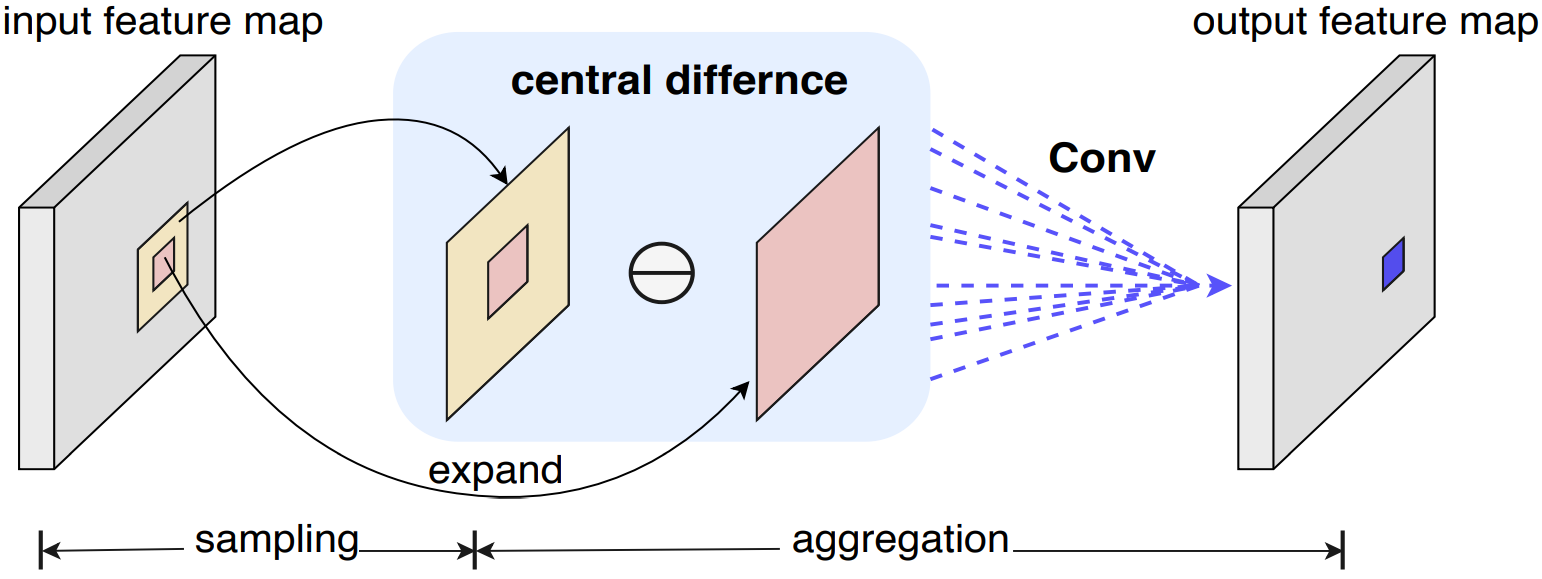Central difference convolution.

y ( p 0 ) = ∑ p n ∈ R w ( p n ) ⋅ ( x ( p 0 + p n ) − x ( p 0 ) ) y({p_0}) = \sum\limits_{{p_n} \in \mathcal{R}} {w({p_n}) \cdot (x(} {p_0} + {p_n})-x(p_0))
p n = ( 0 , 0 ) p_n=(0,0) 时，相对于中心位置 p 0 p_0 本身，梯度值始终等于零

y ( p 0 ) = θ ⋅ ∑ p n ∈ R w ( p n ) ⋅ ( x ( p 0 + p n ) − x ( p 0 ) ) ⏟ central difference convolution + ( 1 − θ ) ⋅ ∑ p n ∈ R w ( p n ) ⋅ x ( p 0 + p n ) ⏟ vanilla convolution y({p_0}) = \theta \cdot \underbrace {\sum\limits_{{p_n} \in \mathcal{R}} {w({p_n}) \cdot (x(} {p_0} + {p_n}) - x({p_0}))}_{{\text{central difference convolution}}} + (1 - \theta ) \cdot \underbrace {\sum\limits_{{p_n} \in \mathcal{R}} {w({p_n}) \cdot x(} {p_0} + {p_n})}_{{\text{vanilla convolution}}}

其中超参数 θ ∈ [ 0 , 1 ] θ∈[0,1] 权衡了intensity-level信息和gradient-level信息之间的贡献。 θ θ 值越高意味中心差分梯度信息越重要。此后，我们将这种广义的中心差分卷积称为CDCCentral Difference Convolution

Implementation for CDC

为了在目前的深度学习框架中实现CDC，我们将CDC卷积公式变形如下
y ( p 0 ) = ∑ p n ∈ R w ( p n ) ⋅ x ( p 0 + p n ) ⏟ vanilla convolution + θ ⋅ ( − x ( p 0 ) ⋅ ∑ p n ∈ R w ( p n ) ) ⏟ central difference term y({p_0}) = \underbrace {\sum\limits_{{p_n} \in \mathcal{R}} {w({p_n}) \cdot x(} {p_0} + {p_n})}_{{\text{vanilla convolution}}} + \underbrace {\theta \cdot ( - x({p_0}) \cdot \sum\limits_{{p_n} \in \mathcal{R}} {w({p_n})} )}_{{\text{central difference term}}}

根据上述变形公式，可以通过PyTorchTensorFlow中的几行代码轻松实现CDC，基于PyTorch的代码在附录A中显示

Relation to Prior Work

在这里，我们讨论CDC香草卷积LBP卷积gabor卷积之间的关系，它们共享相似的设计理念，但着重点不同。在后面的消融研究中将显示CDC在人脸反欺骗任务中的优越性能

Relation to V anilla Convolution CDC是香草卷积的广义形式。从上面的公式可以发现，当设置 θ = 0 \theta=0 时，CDC就变成香草卷积形式，只聚集强度信息而不聚集梯度信息

Relation to Local Binary Convolution 局部二进制卷积（Local Binary ConvolutionLBConv）专注于减少计算量，因此其建模能力受到限制。 CDC专注于无需增添其它任何参数来增强丰富的细节特征表征能力。另一方面，LBConv使用预定义的滤波器来描述局部特征关系，而CDC可以自动学习这些过滤器

Relation to Gabor Convolution Gabor卷积（Gabor convolutionGaborConv致力于增强空间变换（即方向和比例变化）的表示能力，而CDC则更多地致力于表示各种环境中细粒度的鲁棒特征

CDCN

深度图监督的人脸反欺骗方法利用了真假人脸在3D形状中的区别，并为FAS模型提供了逐像素的详细信息以捕获欺骗线索。因此，本文建立了一个类似的深度图监控网络DepthNet作为基准。为了提取更多细粒度和鲁棒的特征以估计面部深度图，引入了CDC来形成中央差分卷积网络（CDCN）。注意到，对于所有CDC算子，当 θ = 0 θ = 0 时，DepthNet是提议的CDCN的特例。

CDCN的细节如下表所示。给定大小为 3 × 256 × 256 3 × 256 × 256 的单个RGB人脸图像，提取多级(低级、中级和高级)融合特征来预测大小为 32 × 32 32 × 32 的灰度人脸深度图。将 θ = 0.7 θ = 0.7 作为默认设置，关于θ的消融研究将在后面给出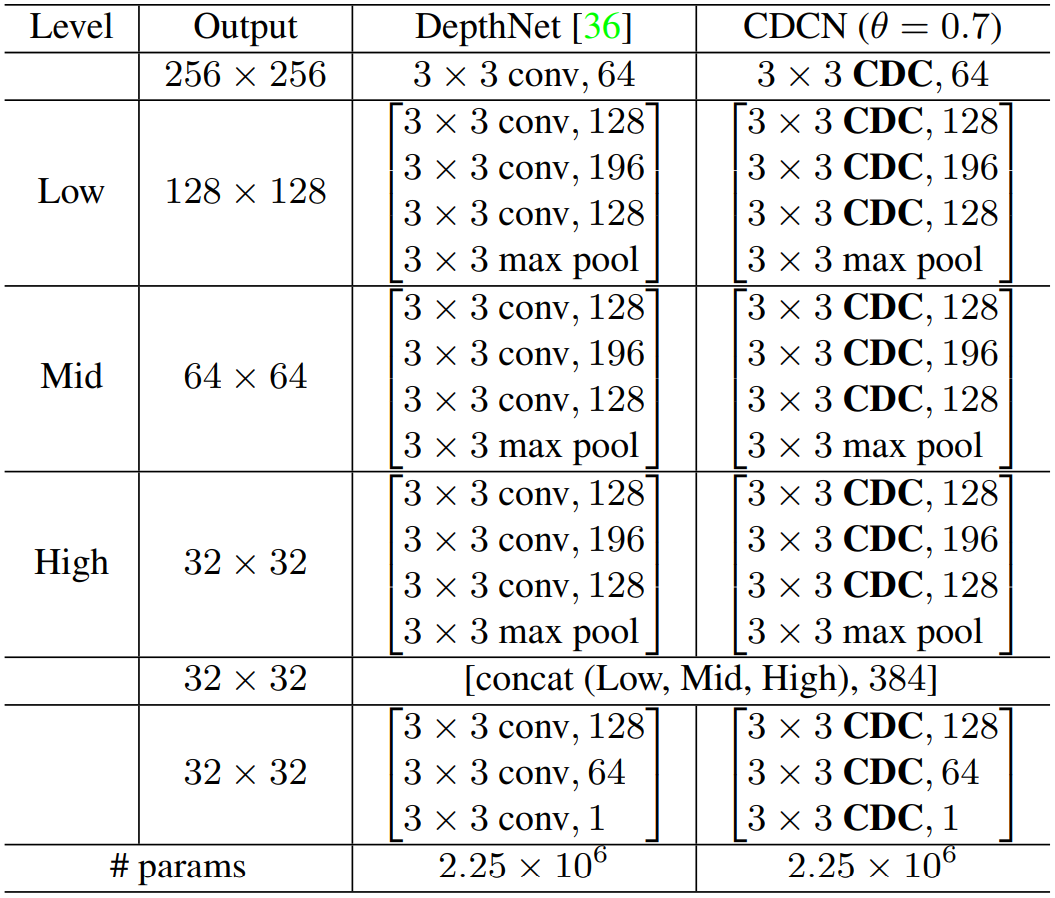Architecture of DepthNet and CDCN. Inside the brackets are the filter sizes and feature dimensionalities. “conv” and “CDC" suggest vanilla and central difference convolution, respectively. All convolutional layers are with stride=1 and followed by a BN-ReLU layer while max pool layers are with stride=2.

对于损失函数，均方误差 L M S E \mathcal{L}_{MSE} 用于像素监督。此外，为了FAS任务对细粒度监督的需求，对比深度损失（Contrastive Depth LossCDL L C D L \mathcal{L}_{CDL} 被认为有助于网络学习更详细的特征。所以整体损失 L o v e r a l l = L M S E + L C D L L_{overall}=\mathcal{L}_{MSE}+\mathcal{L}_{CDL}

CDCN++

从上表中可以看出，CDCN的体系结构被粗略地设计(例如，对于不同level简单地重复相同block)，这对于人脸反欺骗任务可能是次优化的。我们提出了扩展版本CDCN++（如下图所示），它由基于NASbackbone和具有选择性注意能力（selective attention capacity）的多尺度注意力融合模块（Multiscale Attention Fusion Module，MAFM）组成

如下图（a）所示，目标是在三个levelslow-levelmid-levelhigh-level）中搜索cells，以形成用于FAS任务的backbone受人类视觉系统中用于分层组织的专用神经元的启发我们更喜欢自由地搜索这些更灵活和一般化的多级cells（即，具有各种结构的cells）。我们将这种配置命名为“Varied Cells”，并将研究其影响。与以前的工作不同，我们只采用最新输入cell的一个输出作为当前cell的输入

至于cell-level结构，下图（b）展示了每个cell都被表示为 N N 个节点（node { x } i = 0 N − 1 \left\{ x \right\}_{i = 0}^{N - 1} 的有向无环图（directed acyclic graphDAG），其中每个节点表示一个网络层。我们将操作空间表示为 O \mathcal{O} ，下图（c）中展示了八个设计的候选操作（Noneskip-connect捷径连接）和CDCs）。DAG的每条边 ( i , j ) (i,j) 代表从节点 x i x_i 到节点 x j x_j 的信息流，该信息流由结构参数 α ( i , j ) α^{(i,j)} 加权的候选操作组成。特别地，每条边 ( i , j ) (i,j) 可以用一个函数 o ~ ( i , j ) {{\tilde o}^{(i,j)}} 来表示，其中 o ~ ( i , j ) ( x i ) = ∑ o ∈ O η o ( i , j ) ⋅ o ( x i ) {{\tilde o}^{(i,j)}}({x_i}) = \sum\nolimits_{o \in \mathcal{O}} {\eta _o^{(i,j)}} \cdot o({x_i}) 。利用softmax函数将结构参数 α ( i , j ) α_{(i,j)} 放宽为操作权重 o ∈ O o \in \mathcal{O} ，即 η o ( i , j ) = exp ⁡ ( α o ( i , j ) ) ∑ o ′ ∈ O exp ⁡ ( α o ′ ( i , j ) ) \eta _o^{(i,j)} = \frac{{\exp (\alpha _o^{(i,j)})}}{{\sum\nolimits_{o' \in \mathcal{O}} {\exp (\alpha _{o'}^{(i,j)})} }} 。中间节点可表示为 x j = ∑ i < j o ~ ( i , j ) ( x i ) {x_j} = \sum\nolimits_{i < j} {{{\tilde o}^{(i,j)}}({x_i})} ，输出节点 x N − 1 x_{N-1} 由所有中间节点 x N − 1 = ∑ 0 < i < N − 1 β i ( x i ) {x_{N - 1}} = \sum\nolimits_{0 < i < N - 1} {{\beta _i}({x_i})} 的加权和表示。在这里，我们提出了一种节点注意策略来学习中间节点之间的重要性权重 β β ，即 β i = exp ⁡ ( β i ′ ) ∑ 0 < j < N − 1 exp ⁡ ( β j ′ ) {\beta _i} = \frac{{\exp ({\beta _i}^\prime )}}{{\sum\nolimits_{0 < j < N - 1} {\exp ({\beta _j}^\prime )} }} ，其中 β i {\beta _i} 是中间节点 x i x_i 的原始可学习权重 β i ′ {\beta _i}^\prime softmax输出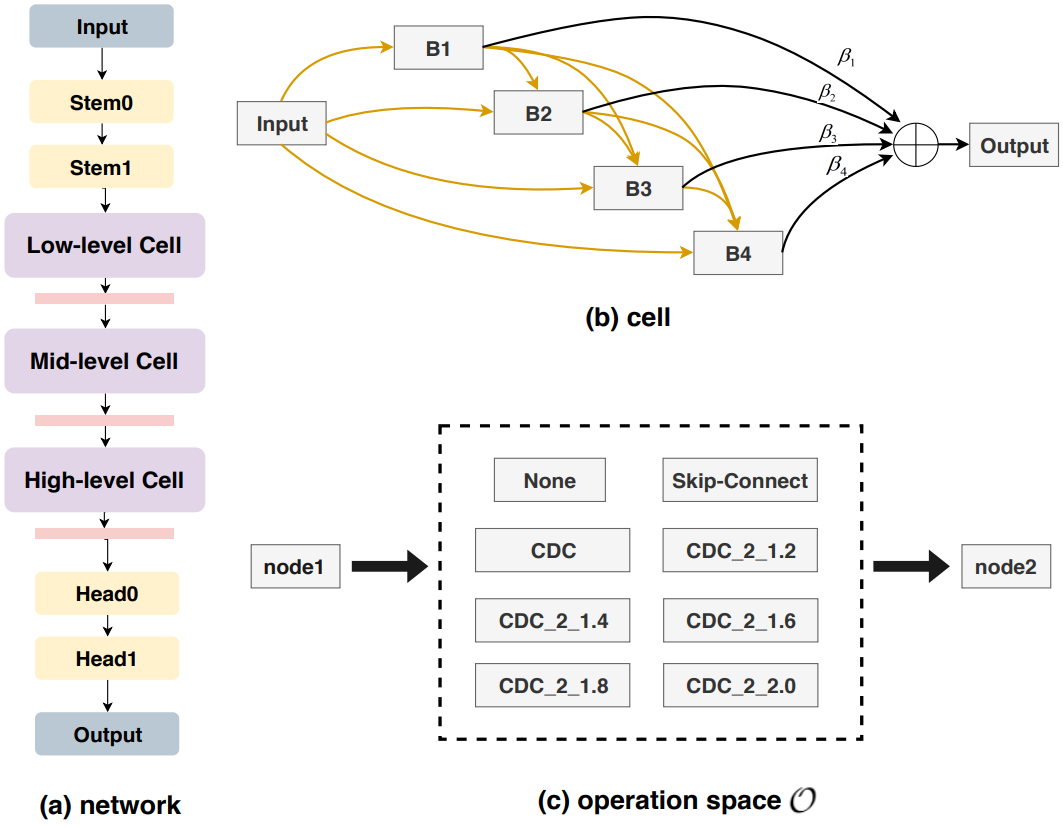Architecture search space with CDC. (a) A network consists of three stacked cells with max pool layer while stem and head layers adopt CDC with 3×3 kernel and θ = 0.7. (b) A cell contains 6 nodes, including an input node, four intermediate nodes B1, B2, B3, B4 and an output node. © The edge between two nodes (except output node) denotes a possible operation. The operation space consists of eight candidates, where CDC_2_r means using two stacked CDC to increase channel number with ratio r first and then decrease back to the original channel size. The size of total search space is 3 × 8 ( 1 + 2 + 3 + 4 ) = 3 × 8 10 3 × 8^{(1+2+3+4)}= 3 × 8^{10}

在搜索阶段， L t r a i n \mathcal{L}_{train} L v a l \mathcal{L}_{val} 分别表示为训练损失和验证损失，它们都是基于深度监督损失 L o v e r a l l \mathcal{L}_{overall} 。网络参数 w w 和结构参数 α α 通过以下bi-level优化问题来学习：
min ⁡ L v a l ( w ∗ ( α ) , α ) , s . t .   w ∗ ( α ) = arg ⁡ min ⁡ w L t r a i n ( w , α ) \min {\mathcal{L}_{val}}({w^*}(\alpha ),\alpha ),\\s.t.{\text{ }}{w^*}(\alpha ) = \arg \mathop {\min }\limits_w {\mathcal{L}_{train}}(w,\alpha )

在收敛之后，最终的分立架构通过以下方式导出：
1）设置 o ( i , j ) = arg ⁡ max ⁡ o ∈ O , o ≠ n o n e p o ( i , j ) {o^{(i,j)}} = \arg {\max _{o \in \mathcal{O},o \ne none}}p_o^{(i,j)}
2）对于每个中间节点，选择一个具有最大 max ⁡ o ∈ O , o ≠ n o n e p o ( i , j ) {\max _{o \in \mathcal{O},o \ne none}}p_o^{(i,j)} 值的输入边；
3)对于每个输出节点，选择具有最大值 max ⁡ 0 < i < N − 1 {\max _{0 < i < N - 1}} 的输入中间节点（表示为“Node Attention”）作为输入。相比之下，选择最后一个中间节点作为输出节点更简单。我们将在后面比较这两种设置

MAFM虽然简单地融合low-mid-high levels特征可以提高搜索到的CDC结构的性能，但是仍然很难找到要关注的重要区域，这不利于学习更多的区分特征。受人类视觉系统选择性注意的启发，不同水平的神经元很可能在它们的感受野中有不同注意力的刺激。在这里，我们提出了一个多尺度注意力融合模块（MAFM），它能够通过空间注意力提炼和融合low-mid-high levelsCDC特征

如下图所示，来自不同level的特征 F \mathcal{F} 通过与kernel size相关的感受野的空间注意力进行细化（即，在我们的例子下，high/semantic level应该的注意力应该具有小的kernel size，而low level的注意力应该具有大的kernel size），然后将它们连接在一起。精炼的特征 F ′ \mathcal{F'} 可如下表示：
F ′ i = F i ⊙ ( σ ( C i ( [ A ( F i ) , M ( F i ) ] ) ) ) , i ∈ { l o w , m i d , h i g h } {{\mathcal{F}'}_i} = {\mathcal{F}_i} \odot (\sigma ({\mathcal{C}_i}([\mathcal{A}({\mathcal{F}_i}),\mathcal{M}({\mathcal{F}_i})]))),i \in \{ low,mid,high\}

其中 ⊙ \odot 代表哈达玛积Hadamard product）。 A \mathcal{A} M \mathcal{M} 分别代表平均池化层和最大池化层。 σ σ 表示sigmoid函数， C \mathcal{C} 代表卷积层。

C l o w \mathcal{C}_{low} C m i d \mathcal{C}_{mid} C h i g h \mathcal{C}_{high} 分别代表 7 × 7 7×7 5 × 5 5×5 3 × 3 3×3 的香草卷积。这里没有选择CDC，因为CDC对全局语义的感知能力有限，而全局语义感知在空间注意力中至关重要。相应的消融研究在后面给出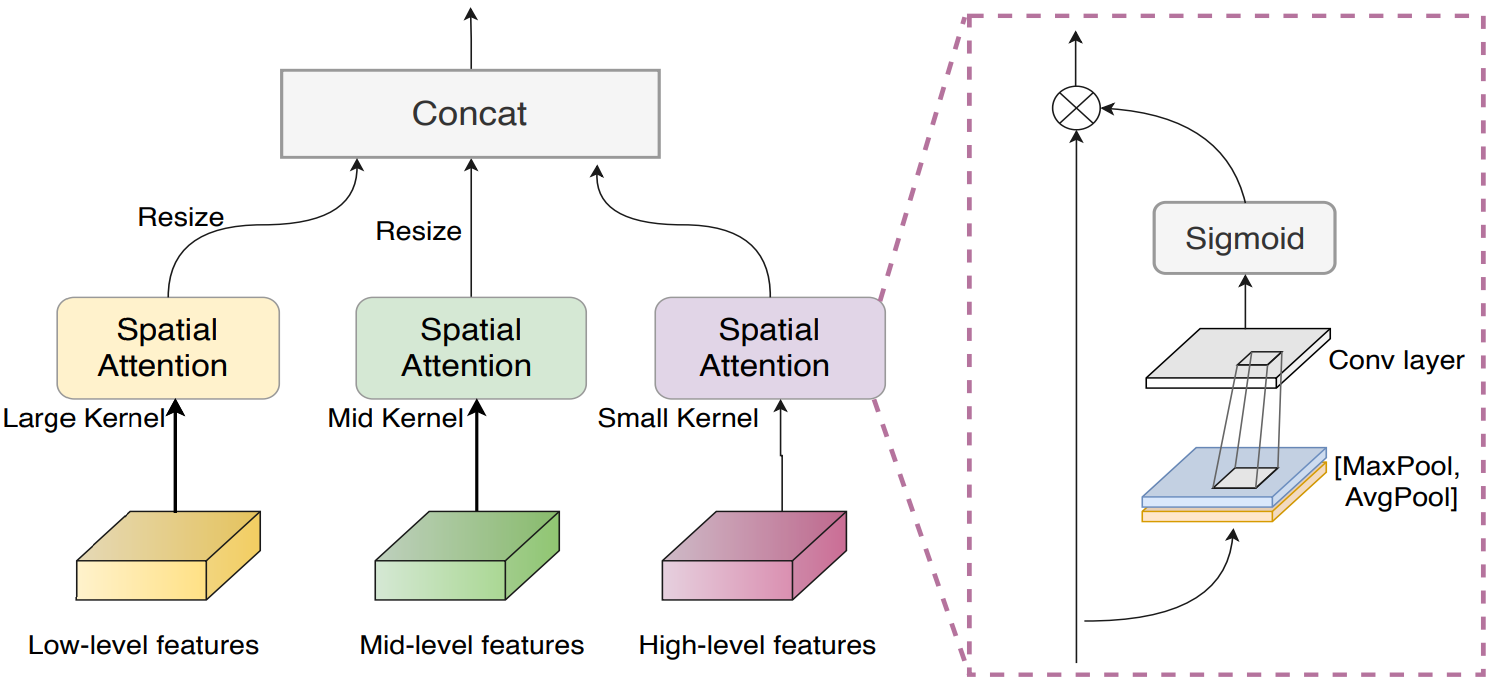Multiscale Attention Fusion Module.

Experiments

在这一部分，进行了大量的实验来证明我们方法的有效性。在下文中，我们将依次描述所采用的数据集和指标（Datasets and Metrics），实施细节（Implementation Details），结果和分析（Results and Analysis

Datasets and Metrics

Databases

本文的实验共使用了六个数据集OULU-NPUSiWCASIA-MFSDReplay-AttackMSU-MFSDSiW-MOULU-NPUSiW是高分辨率数据库，分别包含四个和三个协议用于内部测试来验证模型的泛化（例如，未知的照明和攻击介质）。CASIA-MFSDReplay-AttackMSU-MFSD包含低分辨率视频，其用于交叉测试。SiW-M是为针对未知攻击的跨类型测试而设计的，因为内部有丰富的种攻击类型（SiW-M拥有13种攻击类型）

Performance Metrics

OULU-NPUSiW数据集中，我们遵循其原始的协议和指标（protocols and metrics），即攻击呈现分类错误率Attack Presentation Classification Error RateAPCER）、真实呈现分类错误率Bona Fide Presentation Classification Error RateBPCER）和ACER进行公平比较。CASIA-MFSDReplay-Attack的交叉测试采用半总错误率Half Total Error RateHTER）。AUCArea Under Curve）用于CASIA-MFSDReplay-AttackMSU-MFSD内部数据集的跨类型测试。对于在SiW-M的跨类型型测试，则采用了APCERBPCERACER等差错率Equal Error RateEER

Implementation Details

Depth Generation

本文采用PRNet来为真实活体人脸估计其3D形状并生成尺寸为 32 × 32 32×32 的深度图。为了区分活体人脸和欺骗人脸，在训练阶段，我们将活体人脸的深度图归一化在 [ 0 , 1 ] [0,1] 范围内，同时将欺骗深度图设置为 0 0 矩阵

Training and Testing Setting

Searching Setting

我们采用部分通道连接和边归一化edge normalization）。网络中的初始channel数为 { 32 , 64 , 128 , 128 , 128 , 64 , 1 } \{32,64,128,128,128,64,1\} （如CDCN++图（a）所示），搜索后加倍。训练模型权重时，使用learning rate=1e-4weight decay=5e-5Adam优化器。结构参数使用learning rate=6e-4weight decay=1e-3Adam优化器训练。我们设置batch size=12OULU-NPU的协议 1 1 上搜索了60个eoch，其中架构参数在前10个epoch没有更新。整个进程在三张 1080 Ti 1080\text{Ti} 上进行，共耗时一天

Ablation Study

在本小节中，所有消融研究均基于OULU-NPU的协议 1 1 （训练集和测试集之间的不同的照明条件、环境和位置）进行，以探索我们提出的CDC，CDCN和CDCN++的细节

Impact of θ in CDCN

根据公式
y ( p 0 ) = θ ⋅ ∑ p n ∈ R w ( p n ) ⋅ ( x ( p 0 + p n ) − x ( p 0 ) ) ⏟ central difference convolution + ( 1 − θ ) ⋅ ∑ p n ∈ R w ( p n ) ⋅ x ( p 0 + p n ) ⏟ vanilla convolution y({p_0}) = \theta \cdot \underbrace {\sum\limits_{{p_n} \in \mathcal{R}} {w({p_n}) \cdot (x(} {p_0} + {p_n}) - x({p_0}))}_{{\text{central difference convolution}}} + (1 - \theta ) \cdot \underbrace {\sum\limits_{{p_n} \in \mathcal{R}} {w({p_n}) \cdot x(} {p_0} + {p_n})}_{{\text{vanilla convolution}}}

θ θ 控制着gradient-based的细节贡献，即 θ θ 越高，包含的局部细节信息越多。如下图（a）所示，当 θ ⩾ 0.3 \theta \geqslant 0.3 时，CDC总是获得比香草卷积更好的性能（ θ = 0 ， A C E R = 3.8 % θ = 0，ACER=3.8\% ），表明基于中心差分的细粒度信息对FAS任务是有帮助的。因为在 θ = 0.7 θ = 0.7 时我们获得了最佳性能（ A C E R = 1.0 ACER=1.0% ），所以我们将该设置用于以下实验。除了保持所有层的 θ θ 不变，我们还探索了一种自适应CDC方法来学习每个层的 θ θ ，如附录B所示

CDC vs. Other Convolutions

根据前面的讨论以及CDC和先前卷积之间的关系，我们认为，提出的CDC更适合于FAS任务，由于不同环境中的详细欺骗伪影应该由gradient-based不变特征来表示。下图（b）显示CDC大幅（超过 2 % A C E R 2\%ACER ）优于其他卷积。有趣的是发现LBConv香草卷积表现更好，说明局部梯度信息对于FAS任务很重要**。**GaborConv性能最差，因为它是为捕捉空间不变特征而设计的，这对人脸反欺骗任务没有帮助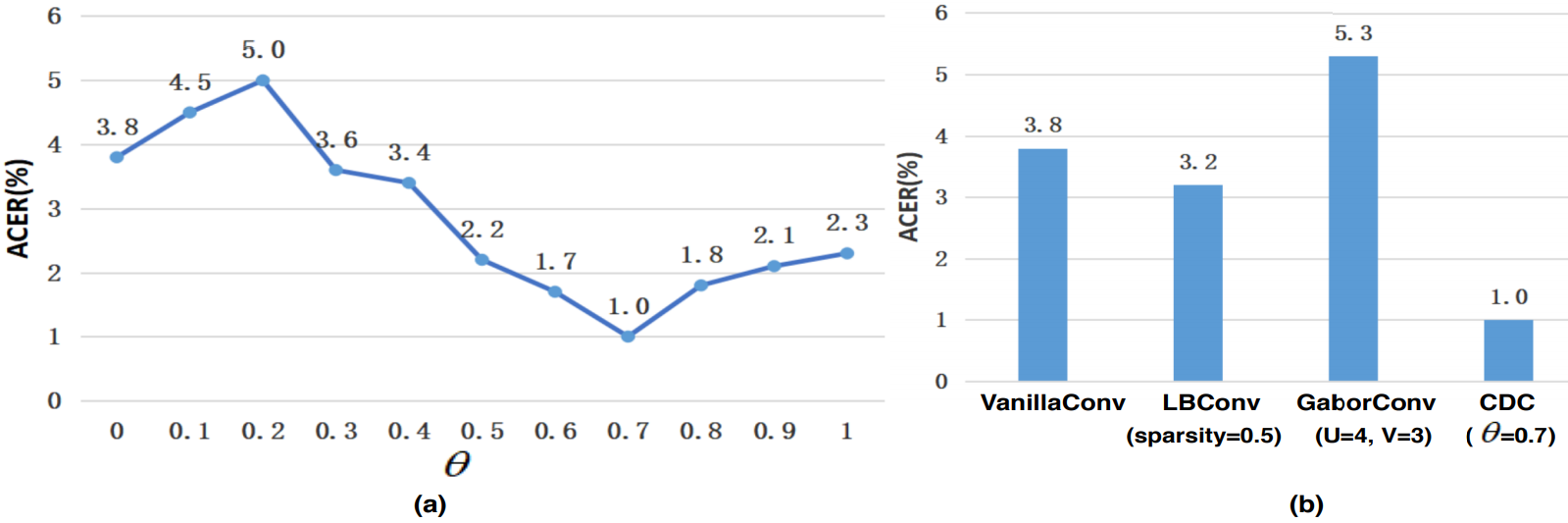(a) Impact of θ in CDCN. (b) Comparison among various convolutions (only showing the hyperparameters with best performance). The lower ACER, the better performance.
Impact of NAS Configuration

下表显示了CDCN++中描述的两种NAS配置的消融研究。即变化的cell和node attention。与具有共享cell和最后一个中间节点作为输出节点的baseline设置相比，这两种配置都可以提高搜索性能。其中有两点原因：

1)在更灵活的搜索约束下，NAS能够找到不同层次的专用cell，这与人类视觉系统更为相似；
2)将最后一个中间节点作为输出可能不是最优的，选择最重要的节点将更为合理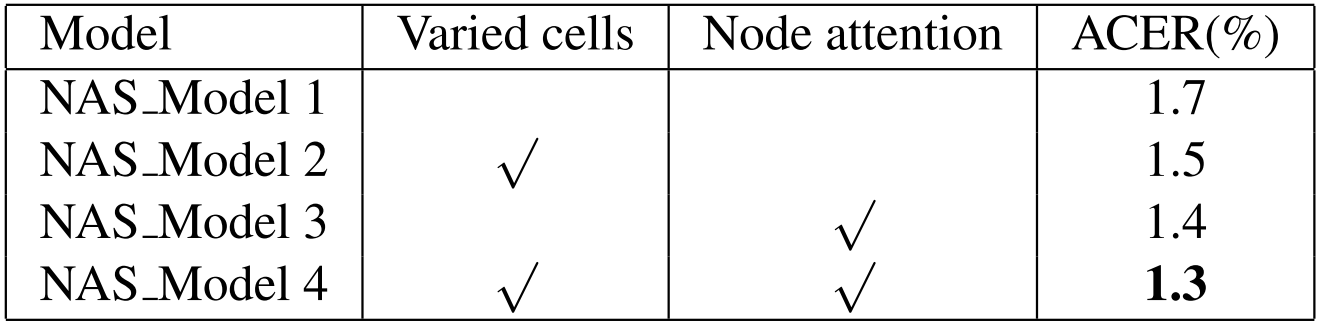The ablation study of NAS configuration.
Effectiveness of NAS Based Backbone and MAFM

下图显示了由基于NASbackboneMAFM组成的CDCN++结构。很明显，来自多个levelcell差异很大，mid-level cell有更深的（四个CDC）层。下表显示了基于NASbackboneMAFM消融研究。从最初的两行可以看出，具有直接多级融合direct multi-level fusion）的基于NASbackbone优于（ 0.3 % A C E R 0.3\% ACER ）不具有NASbackbone，这表明了搜索结构的有效性。与此同时，具有MAFMbackbone比具有直接多级融合结构低 0.5 % A C E R 0.5\% ACER ，这显示了MAFM的有效性。我们还分析了MAFM的卷积类型和kernel size，发现香草卷积更适合捕捉语义空间注意力。除此之外，对于低级和高级特性，注意力内核大小应该分别足够大（ 7 × 7 7×7 ）和足够下小（ 3 × 3 3×3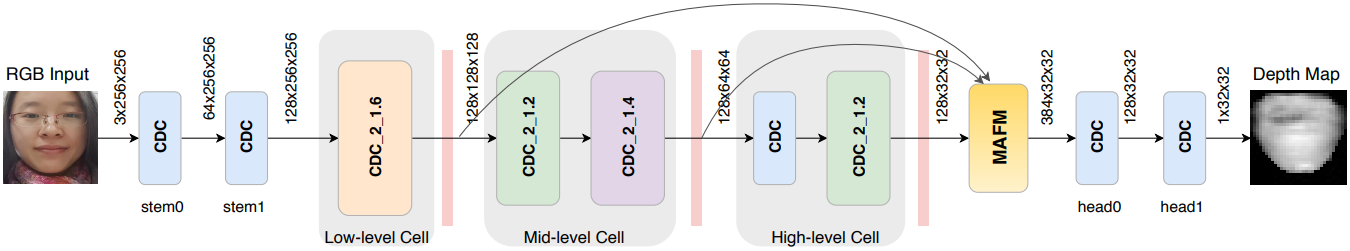The architecture of CDCN++. It consists of the searched CDC backbone and MAFM. Each cell is followed by a max pool layer.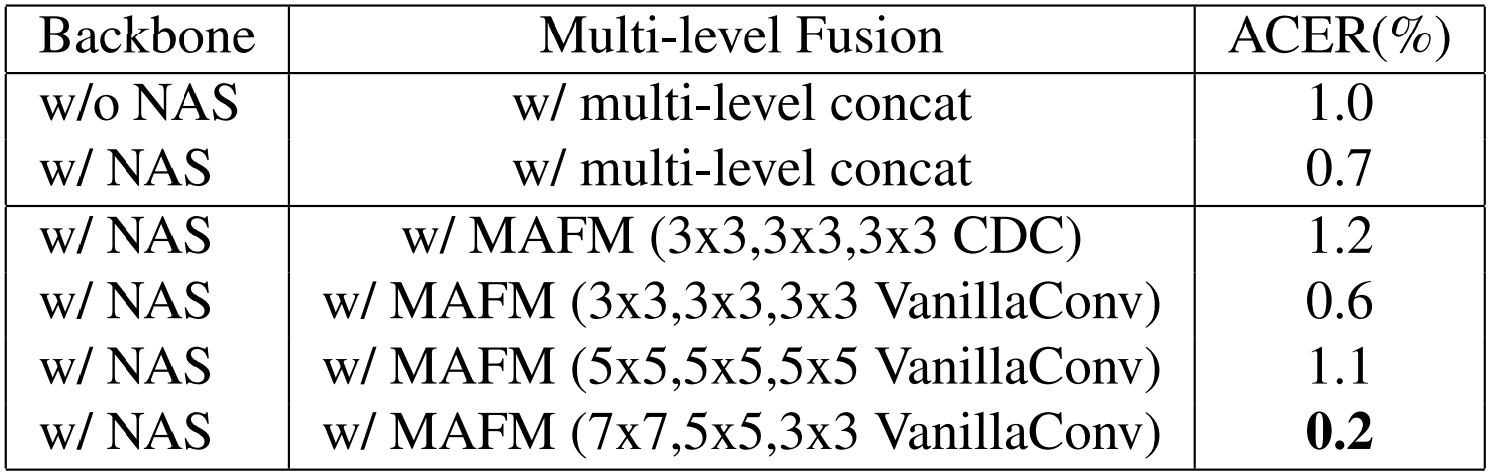The results of intra testing on four protocols of OULU-NPU. We only report the results “STASN ” trained without extra datasets for a fair comparison.

Intra Testing

内部测试在OULU-NPU和SiW数据集上进行。我们严格遵循OULU-NPU的四项协议和SiW的三项协议进行评估。为了公平比较，包括STASN在内的所有用于比较的方法都是在没有额外数据集的情况下训练

Results on OULU-NPU

如下表所示，我们提出CDCN++在所有 4 4 个协议中均排名第一(分别为 0.2 % 0.2\% 1.3 % 1.3\% 1.8 % 1.8\% 5.0 % A C E R 5.0\%ACER )，这表明所提出的方法在外部环境、攻击媒介和输入摄像机差异方面均能得到很好的泛化。与其他最先进的提取多帧动态特征的方法（AuxiliarySTASNGRADIANTFAS-TD）不同，我们的方法只需要frame-level输入，这适更易于现实部署。值得注意的是，CDCN++的基于NASbackbone是可迁移的，并且在所有协议上都可以很好地推广，尽管它是在协议 1 1 上是搜索的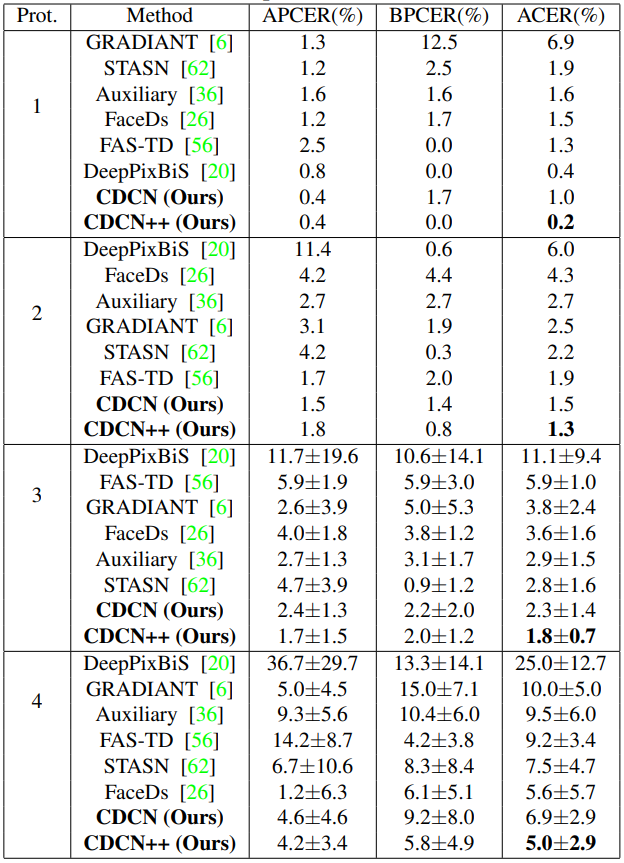The results of intra testing on four protocols of OULU-NPU. We only report the results “STASN ” trained without extra datasets for a fair comparison.
Results on SiW

下表比较了我们的方法与三种最先进的方法AuxiliarySTASNFAS-TDSiW数据集上的性能。从表中可以看出，我们的方法在所有三种协议中均表现最好，揭示了CDC(1)面部姿势和表情的变化，(2)不同欺骗介质的变化，(3)交叉/未知呈现攻击的优异泛化性能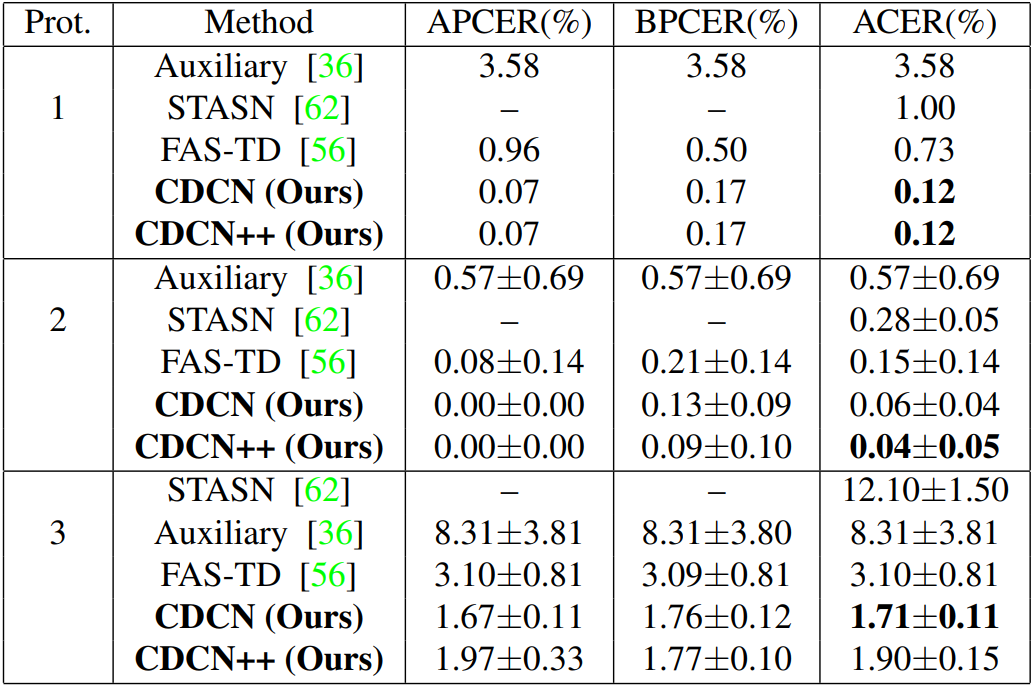The results of intra testing on three protocols of SiW

Inter Testing

为了进一步验证模型的泛化能力，我们分别进行了跨类型和跨数据集测试，以验证模型对未知呈现攻击和未知环境的泛化能力

Cross-type Testing

根据《An anomaly detection approach to face spoofing detection: A new formulation and evaluation protocol.》中提出的协议，我们使用CASIA-MFSDReplay-AttackMSU-MFSD在重放和打印攻击的内部数据集进行跨类型测试。如下表所示，我们提出的基于CDC的方法获得了最佳的整体性能（甚至优于基于零zero-shot学习的方法DTN ），这表明我们在未知攻击中始终具有良好的泛化能力。此外，我们还对最新的SiW-M数据集进行了交叉类型测试，在 13 13 种攻击中取得了最佳的平均 A C E R ACER ( 12.7 % 12.7\% )和 E E R EER ( 11.9 % 11.9\% )。详细结果参见附录三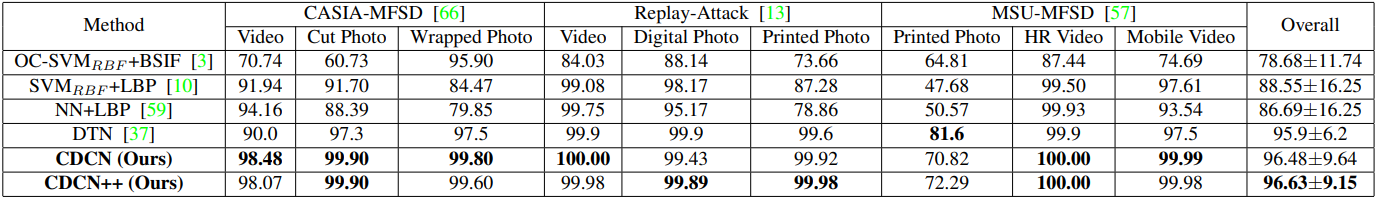AUC (%) of the model cross-type testing on CASIA-MFSD, Replay-Attack, and MSU-MFSD.
Cross-dataset Testing

在这个实验中，有两个跨数据集的测试协议。第一种是在CASIA-MFSD上训练，在Replay-Attack上测试，它被命名为protocol CR；第二种是交换训练数据集和测试数据集，命名为protocol RC。如下表所示，我们提出的CDCN++protocol CR上有 6.5 6.5% H T E R HTER ，以令人信服的 11 11% 的优势优于现有技术。对于protocol RC，我们也优于最先进的frame-level方法（见下表的下半部分）。通过在AuxiliaryFAS-TD中引入类似的时间动态特征，可能进一步提高性能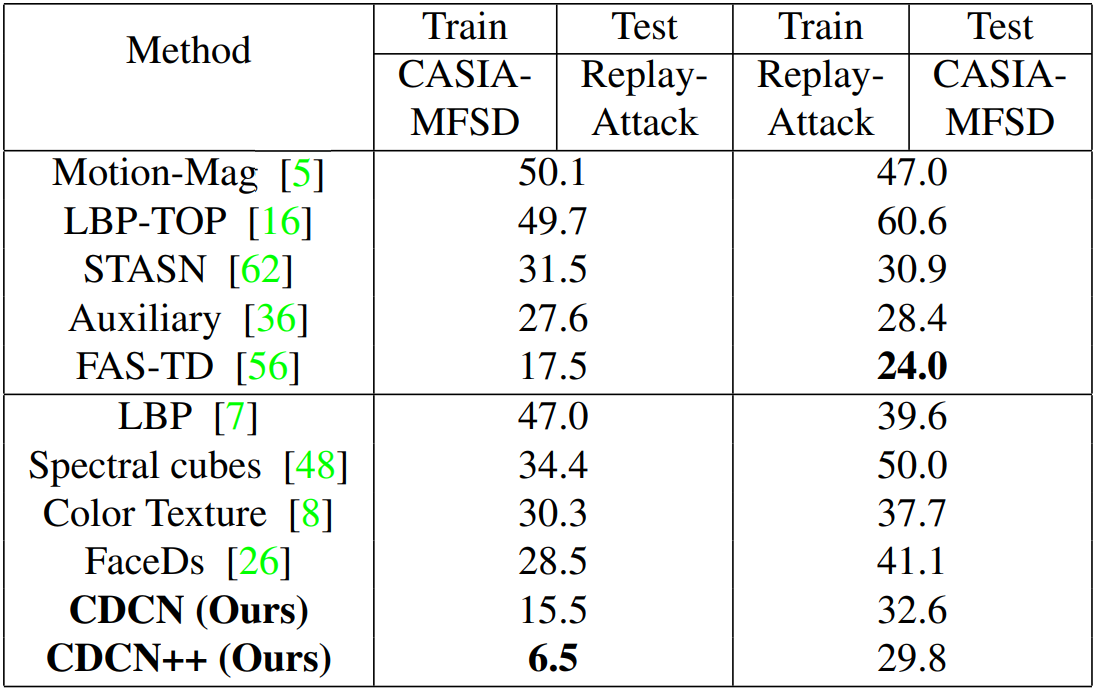The results of cross-dataset testing between CASIA-MFSD and Replay-Attack. The evaluation metric is HTER(%). The multiple-frame based methods are shown in the upper half part while single-frame based methods in bottom half part.

Analysis and Visualization.

在这一小节中，提供了两个视角来展示为什么CDC表现良好

Robustness to Domain Shift

OULU-NPUProtocol-1用于遇到域转移时验证CDC的鲁棒性，即训练/开发和测试集之间的巨大照明差异。下图显示了使用普通卷积的网络在开发集（蓝色曲线）上具有更低 A C E R ACER ，而在测试集（灰色曲线）上具有高 A C E R ACER ，这表明普通卷积在可见域中容易过拟合，但是当光照变化时泛化性较差。相比之下，具有CDC的模型能够在开发（红色曲线）和测试集（黄色曲线）上实现更一致的性能，这表明CDC对域转移具有鲁棒性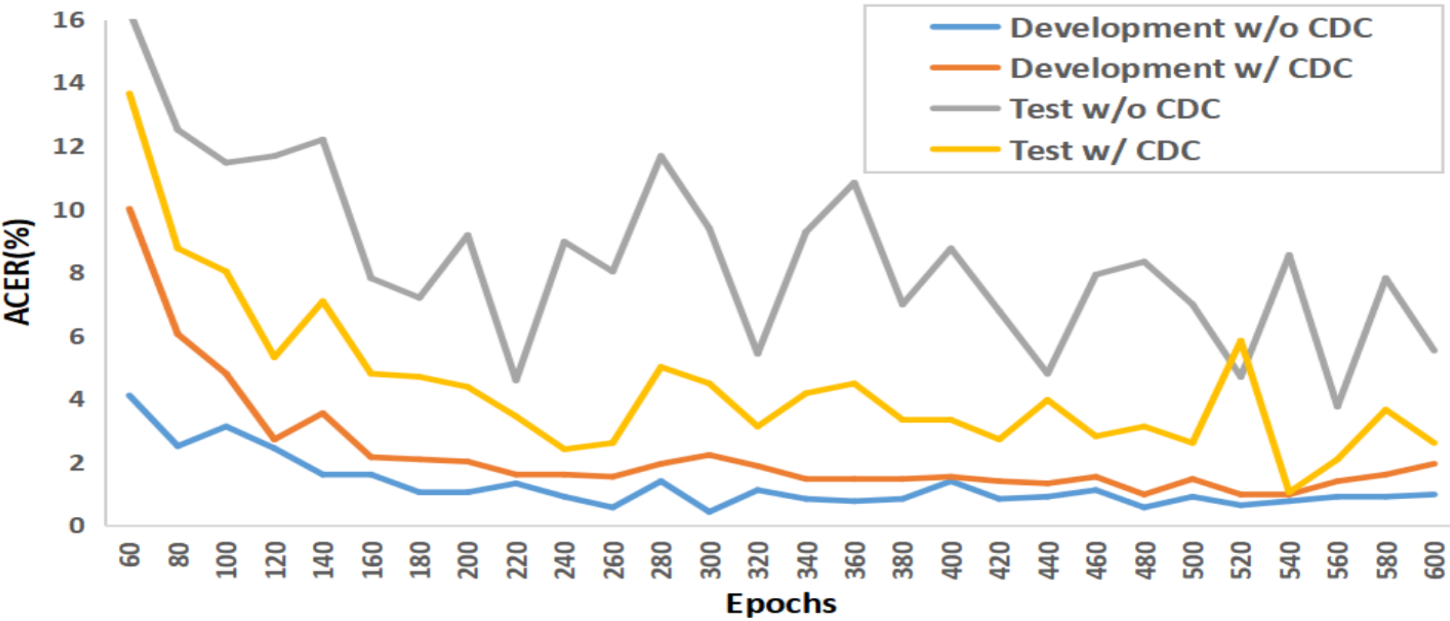The performance of CDCN on development and test set when training on Protocol-1 OULU-NPU.
Features Visualization

下图通过t-SNE展示了测试视频的多级融合特征在OULU-NPU协议 1 1 上的分布。很明显，具有CDC的特征（下图（a））比具有普通卷积的特征（下图（b））呈现出更好的聚类行为，这证明了CDC区分活脸和欺骗脸的能力。特征图（不具有或具有CDC）和MAFM的注意力图的可视化可在附录D中找到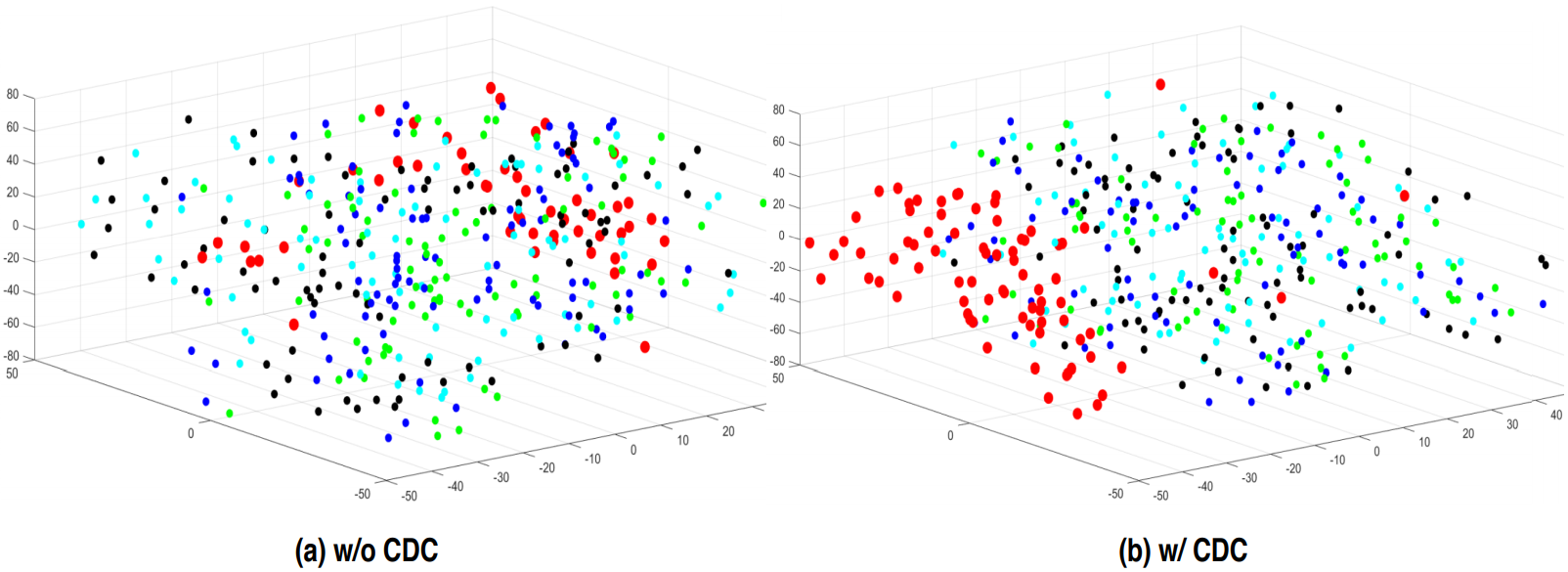3D visualization of feature distribution. (a) Features w/o CDC. (b) Features w/ CDC. Color code used: red=live, green=printer1, blue=printer2, cyan=replay1, black=replay2.

Conclusions and Future Work

本文提出了一种新的用于人脸反欺骗任务的算子——中心差分卷积Central Difference ConvolutionCDC）。基于CDC，设计了一种中心差分卷积网络（CDCN）。我们还提出了由搜索的CDC backbone多尺度注意力融合模块MAFM）组成的CDCN++结构。大量实验验证了所提方法的有效性。我们注意到CDC的研究仍处于早期阶段。未来的方向包括：
1)为每一层/通道设计上下文感知的自适应CDC；
2)探索其他属性（例如，域泛化domain generalization））和在其他视觉任务上的适用性（例如，图像质量评估和人脸伪造检测FaceForensics））

Acknowledgment

This work was supported by the Academy of Finland for project MiGA (grant 316765), ICT 2023 project (grant 328115), and Infotech Oulu. As well, the authors wish to acknowledge CSC IT Center for Science, Finland, for computational resources.

Appendix

A. Derivation and Code of CDC

下图展示了详细的CDC推导公式，并给出了CDC的PyTorch代码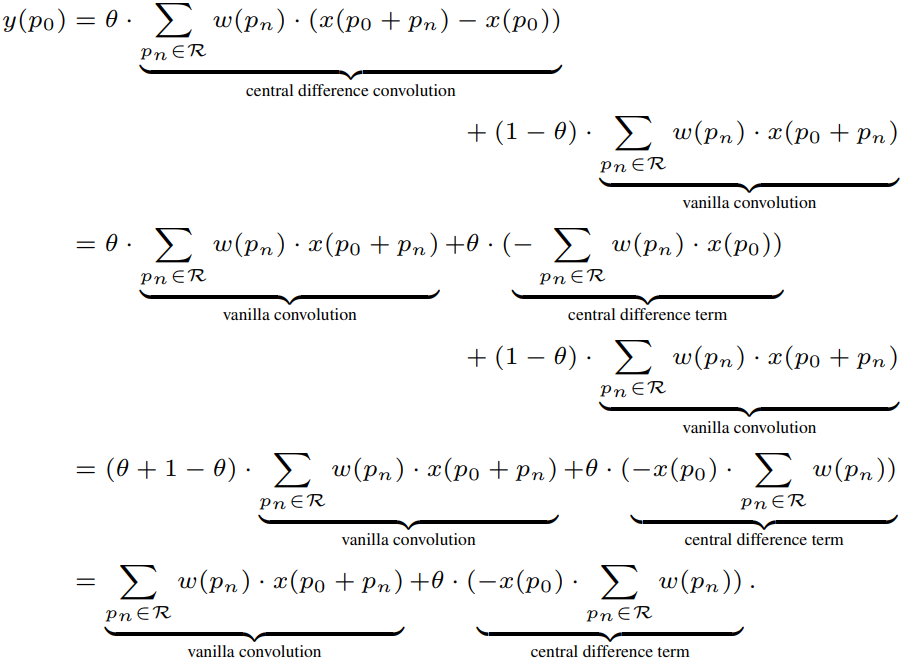import torch.nn as nn
import torch.nn.functional as F

class CDC(nn.Module):
def __init__(self, IC, OC, K=3, P=1, theta=0.7):
# IC, OC: in_channels, out_channels
# theta: hyperparameter in CDC
super(CDC, self).__init__()
self.vani = nn.Conv2d(IC, OC, kernel_size=K, padding=P)
self.theta = theta

def forward(self, x):
# x: input features with shape [N,C,H,W]
out_vanilla = self.vani(x)

kernel_diff = self.vani.weight.sum(2).sum(2)
kernel_diff = kernel_diff[:, :, None, None]

return out_vanilla - self.theta * out_CD

B. Adaptive θ θ for CDC

虽然人脸反欺骗任务可以手动测量最佳超参数 θ = 0.7 θ = 0.7 ，但在将中心差分卷积(CDC)应用于其他数据集/任务时，寻找最合适的 θ θ 仍然很麻烦。这里，我们将 θ θ 视为每一层数据驱动的可学习权重。一个简单的实现是利用 S i g m o i d ( θ ) Sigmoid(θ) 来保证输出范围在 [ 0 , 1 ] [0,1] 以内

如下图所示，可以发现有趣的是在低（第 2 2 层至第 4 4 层）和高（第 8 8 层至第 10 10 层）level中学习的权重值相对较小，而在中（第 5 5 层至第 7 7 层）level中学习的权重值较大。这表明中心差分梯度信息对于中层（mid-level）特征可能更重要。就性能比较而言，从下图（b）中可以看出，使用常数 θ = 0.7 θ = 0.7 CDC，自适应CDC获得了可比拟的结果（ 1.8 % 1.8\% 1.0 % A C E R 1.0\% ACER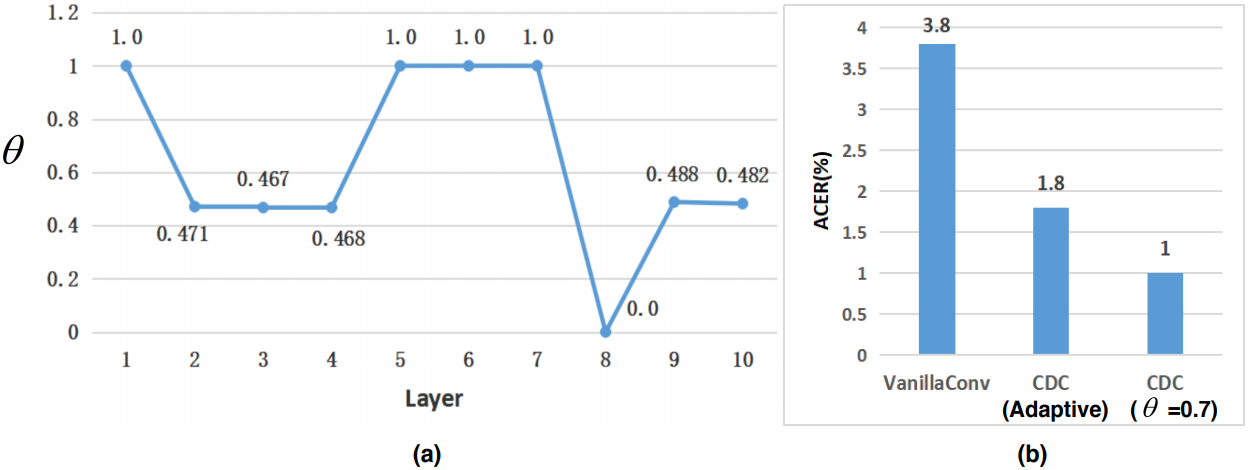Adaptive CDC with learnable θ for each layer. (a) The learned θ weights for the first ten layers. (b) Performance comparison on Protocol-1 OULU-NPU.

C. Cross-type Testing on SiW-M

SiW-M数据集上遵循相同的交叉类型测试协议（13 attacks leave-one-out），我们将我们提出的方法与三个最近的人脸反欺骗方法进行比较，以验证未知道攻击的泛化能力。如下表所示，我们的CDCN++总体上实现了更好的 A C E R ACER E E R EER ，分别比以前的先进水平提高了 24 % 24\% 26 % 26\% 。具体来说，我们检测到几乎所有的“Impersonation”和“Partial Paper”攻击( E E R = 0 % EER=0\% )，而以前的方法在“Impersonation”攻击上表现不佳。很明显，我们大幅降低了掩模攻击(“HalfMask”、“SiliconeMask”、“TransparentMask”和“MannequinHead”)的 E E R EER A C E R ACER ，这表明我们基于CDC的方法对3D非平面攻击得到了很好的泛化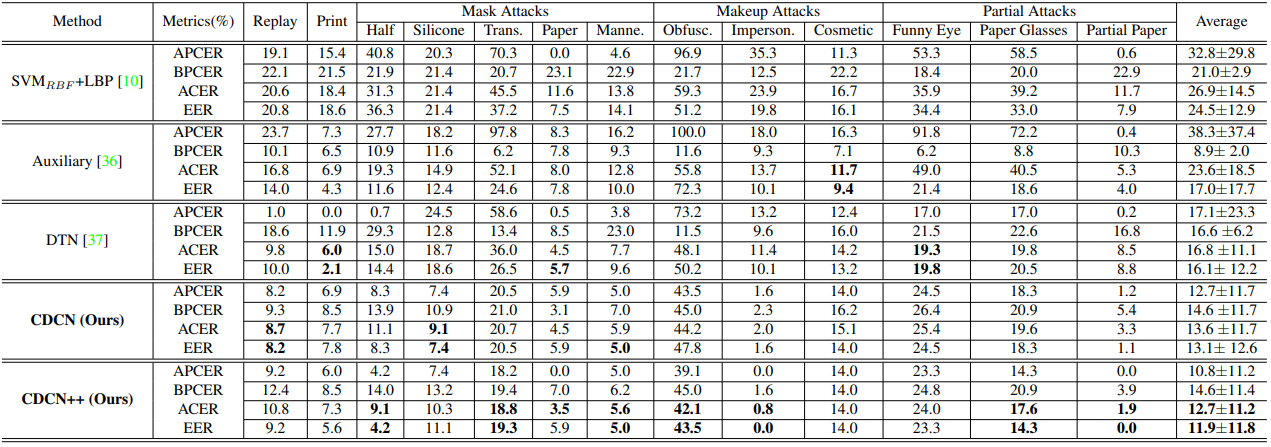The evaluation and comparison of the cross-type testing on SiW-M

D. Feature Visualization

MAFM的低层特征和相应的空间注意力图在下图中可视化。很明显，真实活体脸和欺骗脸的特征和注意力图都有很大的不同
1)对于低级特征(参见下图第2和第3行)，来自欺骗面部的神经激活在面部和背景区域之间似乎比来自活体面部的更均匀。值得注意的是，带有CDC的特性更有可能捕获详细的欺骗模式(例如，“Print1”中的晶格伪影和“Replay2”中的反射伪影)；

2)对于MAFM的空间注意力图(见下图第4行)，头发、面部和背景的所有区域对于活体面部具有相对较强的激活，而面部区域对于欺骗面部贡献较弱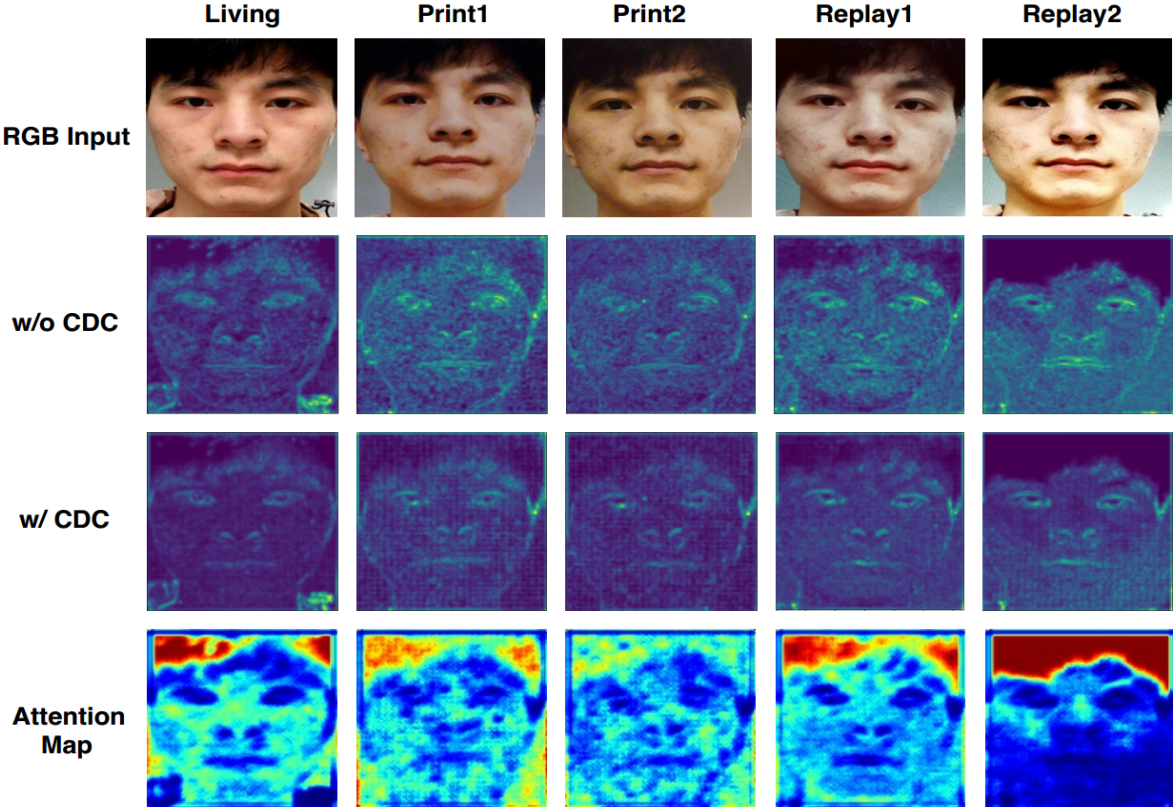Features visualization on living face (the first column) and spoofing faces (four columns to the right). The four rows represent the RGB images, low-level features w/o CDC, w/ CDC and low-level spatial attention maps respectively. Best view when zoom in.

资源链接

展开全文神经网络 人脸反欺骗
• #合并不同项，并复制给新变量d d=a.symmetric_difference(b) print(d) #合并不同项，并更新自己 a.symmetric_difference_update(b) print(a) 2.三元运算(为真时的结果 if 判定条件 else 为假时的结果) #说明，if判断...

1.set是一个无序且不重复的元素集合。

集合对象是一组无序排列的可哈希的值，集合成员可以做字典中的键。集合支持用in和not in操作符检查成员，由len()内建函数得到集合的基数(大小)， 用 for 循环迭代集合的成员。但是因为集合本身是无序的，不可以为集合创建索引或执行切片(slice)操作，也没有键(keys)可用来获取集合中元素的值。

set和dict一样，只是没有value，相当于dict的key集合，由于dict的key是不重复的，且key是不可变对象因此set也有如下特性：

1)不重复

2)元素为不可变对象

#!/usr/bin/env python

# -*- coding:utf-8 -*-

a={11,22,33,24,8}

b={24,50,88,8}

c={24,8}

print(a)

#增加新的元素

print(a)

#清空集合

a.clear()

#找a中存在,b中不存在的集合,并将其赋值给新变量c

c=a.difference(b)

print (c)

# 找a中存在，b中不存在的集合，并更新自己

a.difference_update(b)

print(a)

#remove移除元素，不存在的元素报错

a.remove(11)

#pop随机移除集合中的某个元素

a.pop()

print(a)

#两个集合的交集,intersection是将交集赋予给新的变量ret，intersection_update是取交集并更新自己

ret=a.intersection(b)

a.intersection_update(b)

print (ret)

print(a)

#判断c和a是否不存在交集(有交集False,无交集True)

print(c.isdisjoint(a))

#判断c是否是a的子集

print(c.issubset(a))

#判断c是否是a的父集

print(c.issuperset(a))

#合并集合a和b，得到的值覆盖a

a.update(b)

print(a)

#增加迭代

a.update([1,2,3])

print(a)

#合并不同项，并复制给新变量d

d=a.symmetric_difference(b)

print(d)

#合并不同项，并更新自己

a.symmetric_difference_update(b)

print(a)

2.三元运算(为真时的结果 if 判定条件 else 为假时的结果)

#说明，if判断条件为真时，name='kobe',否则name='byrant'

name='kobe' if 2>3 else "byrant"

print(name)3.赋值，深浅拷贝定义

直接赋值：其实就是对象的引用(别名)。

浅拷贝(copy)：拷贝父对象，不会拷贝对象内部的子对象。

深拷贝(deepcopy)： copy 模块的 deepcopy 方法，完全拷贝了父对象及其子对象。

#数字和字符串的赋值和深浅拷贝之后，内存地址都一样

1 importcopy2 n1='kobe'

3 n2=n14 print(id(n1))5 print(id(n2))6 n3=copy.copy(n1)7 print(id(n3))8 n4=copy.deepcopy(n1)9 print(id(n4))

#列表，字典赋值之后内存地址一样，深浅拷贝之后，内存地址不一样

import copy

l1=['kobe',{'k1':8,"k2":24},10]

print(l1,id(l1))

l2=l1

print(l2,id(l2))

l3=copy.copy(l1)

print(l3,id(l3))

l4=copy.deepcopy(l1)

print(l4,id(l4))

#例子:

alist=[1,2,3,["a","b"]]

import copy

a=alist

c=copy.copy(alist)

d=copy.deepcopy(alist)

print (alist) #[1, 2, 3, ['a', 'b']]

print(a) #[1, 2, 3, ['a', 'b']]

print (c) #[1, 2, 3, ['a', 'b']]

print(d) #[1, 2, 3, ['a', 'b']]

alist.append(8) #列表中增加一个元素

print (alist) #[1, 2, 3, ['a', 'b'], 8]

print(a) #赋值:[1, 2, 3, ['a', 'b'], 8] 也随着增加了元素

print (c) #浅拷贝:[1, 2, 3, ['a', 'b']] 没有拷贝增加的元素

print(d) #深拷贝：[1, 2, 3, ['a', 'b']] 没变

alist.append("c") #列表中第三个元素中再追加一个元素

print(alist) #[1, 2, 3, ['a', 'b', 'c'], 8]

print(a) #[1, 2, 3, ['a', 'b', 'c'], 8] 也随着增加了元素

print(c) #浅拷贝:[1, 2, 3, ['a', 'b', 'c']] 里面子对象中增加了一个元素

print(d) #深拷贝:[1, 2, 3, ['a', 'b']] 没变

#结论:

# 赋值:随着原始数据的改变而改变.

# 浅拷贝:只拷贝第一层的父对象，不拷贝子对象，原始数据的子对象中的元素改变，浅拷贝里子对象的元素也会改变.

# 深拷贝:原始对象的改变不会造成深拷贝里任何子元素的改变.

展开全文• Pixel Difference Convolution（PDC） 3. PiDiNet 4. 结果展示 5. 差分卷积代码实现 5.1 CPDC 5.2 APDC 5.3 RPDC 参考文献： Su, Z., Liu, W., Yu, Z., Hu, D., Liao, Q., Tian, Q., ... & Liu, L. (2021). Pixel ...

参考文献： Su, Z., Liu, W., Yu, Z., Hu, D., Liao, Q., Tian, Q., ... & Liu, L. (2021). Pixel Difference Networks for Efficient Edge Detection. In Proceedings of the IEEE/CVF International Conference on Computer Vision (pp. 5117-5127).

文章链接：
https://openaccess.thecvf.com/content/ICCV2021/papers/Su_Pixel_Difference_Networks_for_Efficient_Edge_Detection_ICCV_2021_paper.pdf

摘要

motivations:
基于CNN的边缘检测的高性能是通过一个大的预训练CNN主干来实现的，该主干占用大量内存和能量。传统边缘检测器（如Canny、Sobel和LBP）很少被研究。
contributions:
提出了一种简单、轻量级但有效的架构，称为像素差分网络（PiDiNet），用于有效的边缘检测。PiDiNet采用了新颖的像素差卷积，将传统的边缘检测算子集成到现代CNN中流行的卷积运算中，以增强任务性能。
experiments:
在BSDS500、NYUD和Multicue上进行了大量的实验，以证明其有效性、高训练和推理效率。当仅使用BSDS500和VOC数据集从头开始训练时，PiDiNet可以超过BSDS500数据集上记录的人类感知结果（在ODS F-measure中为0.807 vs 0.803），速度为100 FPS，参数小于1M。参数小于0.1M的更快版本的PiDiNet仍然可以以200 FPS的速度实现相当的性能。NYUD和Multicue数据集的结果显示了类似的观察结果。

1. Introduction

直观地说，边缘表现出不同的特定模式，如直线、拐角和“X”连接。一方面，传统的边缘算子受到这些直觉的启发（下图）。基于梯度计算通过显式计算像素差来编码用于边缘检测的重要梯度信息。然而，这些手工制作的边缘算子或基于学习的边缘检测算法由于其浅层结构通常不够强大。
另一方面，CNN可以学习丰富的分层图像表示，其中普通CNN内核用作探测局部图像模式。然而，CNN核是从随机初始化的，它没有对梯度信息进行显式编码，这使得它们很难聚焦于边缘相关的特征。基于CNN的实现边缘检测的缺点还包括：
模型尺寸大、内存消耗大、计算成本高、运行效率低、吞吐量低、标签效率低，需要对大规模数据集进行模型预训练。

设计了一种新的卷积运算，以满足以下需要。首先，它可以很容易地捕获图像梯度信息，便于边缘检测，并且CNN模型可以更专注于处理大量不相关的图像特征。其次，深层CNN强大的学习能力仍然可以保留，以提取语义上有意义的表示，从而实现鲁棒和准确的边缘检测。在本文中，我们提出了像素差卷积（PDC），首先计算图像中的像素差，然后与核权重卷积以生成输出特征（见图3）。PDC效果如下图所示：2. Pixel Difference Convolution（PDC）

像素差分卷积与普通的vanilla卷积的区别仅在于前者对像素对的差值做卷积，而后者对单个像素值做卷积。公式如下所示：在具体解释PDC之前，需要扯一嘴LBP是什么东西。

LBP（Local Binary Pattern，局部二值模式）是一种用来描述图像局部纹理特征的算子；它具有旋转不变性和灰度不变性等显著的优点。它是首先由T. Ojala, M.Pietikäinen, 和D. Harwood 在1994年提出(注意与本文所属一所大学)，用于纹理特征提取。而且，提取的特征是图像的局部的纹理特征；
计算方法：
原始的LBP算子定义为在33的窗口内，以窗口中心像素为阈值，将相邻的8个像素的灰度值与其进行比较，若周围像素值大于中心像素值，则该像素点的位置被标记为1，否则为0。这样，33邻域内的8个点经比较可产生8位二进制数（通常转换为十进制数即LBP码，共256种），即得到该窗口中心像素点的LBP值，并用这个值来反映该区域的纹理信息。如下图所示：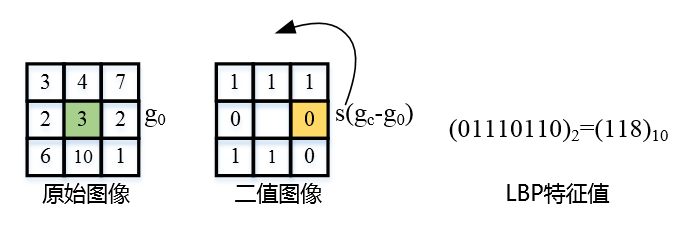LBP的相关扩展：将上述LBP的计算范围扩展以解决3×3邻域模板过小，无法捕获大尺度纹理结构的缺点，即下图所示：
①Circle LBPLBPROT：在Circle LBP的基础上，解决模板具有旋转不变性的问题。

PDC是将上述LBP算法与CNN进行结合，定义了三种PDC的计算方式：central PDC (CPDC), angular PDC(APDC) and radial PDC (RPDC)。如下图所示：• APDC计算示意图与证明:• CPDC计算示意图与证明:• RPDC计算示意图与证明:需要注意的是，基于以上公式，一旦模型训练好了，就可以先计算模板权重的差值，再基于权重与像素点进行卷积运算，从而加快推理速度。
这也是这个论文比较大的创新点所在：

在训练阶段运用两倍的算力训练差分卷积的效果，而推理阶段的速度与普通卷积神经网络一致。

3. PiDiNet

网络是作者自己设计的轻量型网络，大量用了可分离卷积，通道注意力结构和并联膨胀卷积提取特征。从头开始训练，没有调用预训练模型。
以及作者后续做了很多消融实验调整模型参数。值得提一句这里的损失函数，用的是 annotator-robust loss function，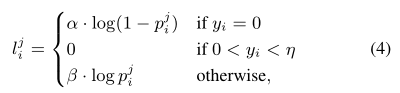where y i is the ground truth edge probability, η is a predefined threshold, meaning that a pixel is discarded and not considered to be a sample when calculating the loss if it is marked as positive by fewer than η of annotators to avoid confusing, β is the percentage of negative pixel samples and
α = λ · (1 − β).

最终损失函数如下：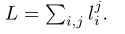4. 结果展示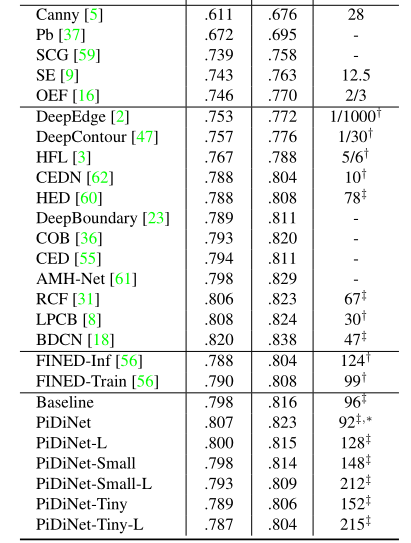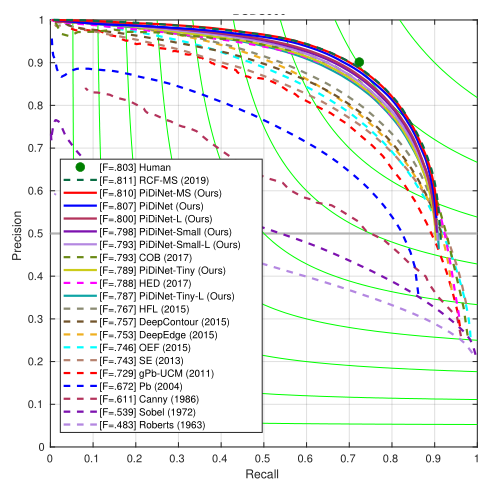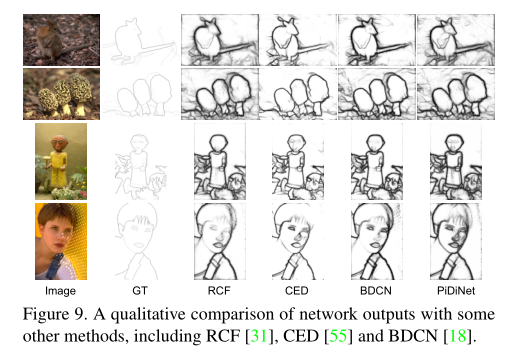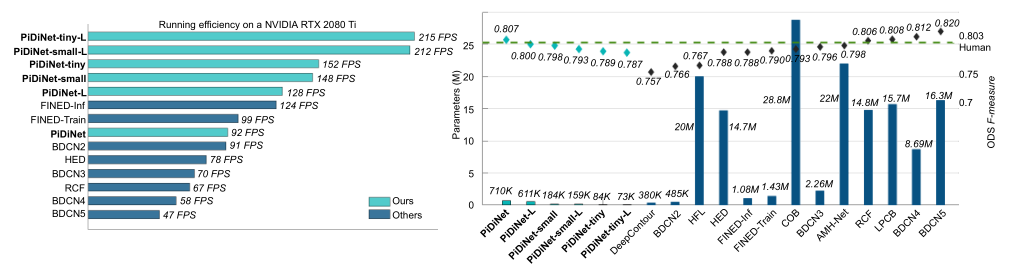5. 差分卷积代码实现

5.1 CPDC

基于第三节的公式（3），CPDC差分卷积将转为普通卷积相减：

def func(x, weights, bias=None, stride=1, padding=0, dilation=1, groups=1):
assert dilation in [1, 2], 'dilation for cd_conv should be in 1 or 2'
assert weights.size(2) == 3 and weights.size(3) == 3, 'kernel size for cd_conv should be 3x3'

weights_c = weights.sum(dim=[2, 3], keepdim=True) #B,C,3,3 -> B,C,1,1
yc = F.conv2d(x, weights_c, stride=stride, padding=0, groups=groups)
return y - yc

5.2 APDC

基于第三节的公式（7），APDC差分卷积将转为普通卷积相减：

def func(x, weights, bias=None, stride=1, padding=0, dilation=1, groups=1):
assert dilation in [1, 2], 'dilation for ad_conv should be in 1 or 2'
assert weights.size(2) == 3 and weights.size(3) == 3, 'kernel size for ad_conv should be 3x3'

shape = weights.shape
weights = weights.view(shape, shape, -1) #B,C,3,3 -> B,C,9
weights_conv = (weights - weights[:, :, [3, 0, 1, 6, 4, 2, 7, 8, 5]]).view(shape) # clock-wise
return y

5.3 RPDC

基于第三节的公式（9），APDC差分卷积将转为普通卷积相减：

def func(x, weights, bias=None, stride=1, padding=0, dilation=1, groups=1):
assert dilation in [1, 2], 'dilation for rd_conv should be in 1 or 2'
assert weights.size(2) == 3 and weights.size(3) == 3, 'kernel size for rd_conv should be 3x3'

shape = weights.shape
if weights.is_cuda:
buffer = torch.cuda.FloatTensor(shape, shape, 5 * 5).fill_(0)
else:
buffer = torch.zeros(shape, shape, 5 * 5)
weights = weights.view(shape, shape, -1) #B,C,5,5 -> B,C,25
buffer[:, :, [0, 2, 4, 10, 14, 20, 22, 24]] = weights[:, :, 1:]
buffer[:, :, [6, 7, 8, 11, 13, 16, 17, 18]] = -weights[:, :, 1:]
buffer[:, :, 12] = 0
buffer = buffer.view(shape, shape, 5, 5)
return y

展开全文深度学习 edge
• C++set_difference的使用方式 功能描述： 求两个集合的差集 函数原型： //set_union(iterator beg1, iterator end1, iterator beg2, iterator end2, iterator dest); * //求两个集合的交集 * //beg1容器1开始...c++ stl C++算法
• difference的功能 difference的用法 什么是差集 a，b两个集合，由所有属于a且不属于b的元素组成的集合叫做a与b的差集 difference的功能 返回集合的差集，即返回的集合元素包含在第一个集合中，但不包含在第二个...python 集合
• " + "first difference at " + index + ", difference of length " + diffLength + ", \"" + difference + "\"" + "\ngolden:\n" + golden + "\nnew:\n" + results + "\ndiffChars g='" + golden.charAt(index) + ...
• Set newSet = Sets.difference(set1, set2); System.out.println(newSet); } 以set1为基础,筛选出set2中不存在的 结果:[ 4 , 5 ] 如果set2中包含set1中没有的,则会被忽略,如set中有 6 ,则输出仍然是[ 4 , 5 ],...java ajax sql
• Python集合(set)合并教程Python集合(set)合并方法比较函数描述symmetric_difference使用 symmetric_difference 函数，合并两个集合时，合并的结果会通过返回值的形式来返回。symmetric_difference_update使用 ...
• 精品视频课程推荐Java Web开发理论部分视频教程系统掌握开发实际的Java Web应用所需的理论知识和技能(Servlet、Jsp、JavaBean、TagLib、EL、JSTL、MVC模式、连接池DataSource、JNDI等知识)。涉及项目：在线投票计数...
• 1.1 short-term TDM TDM全名为 temporal difference module，可以知道TDM模块里存在一些差值的计算，而short-term指的就是短时间内局部信息的差值。举例说明，对于给定的一个视频V来说，首先类似TSN的处理方式，将...
• #合并不同项，并复制给新变量d d=a.symmetric_difference(b) print(d) #合并不同项，并更新自己 a.symmetric_difference_update(b) print(a) 2.三元运算(为真时的结果 if 判定条件 else 为假时的结果) #说明，if判断...
• difference_type 带符号整数类型，足够保存两个迭代器之间的距离 difference_type使用来表示两个迭代器之间的距离的。 vector<int> v(10, 1); vector<int>::difference_type len = v.begin() - v.end(); vector...
• set(['Tom','Lucy','Ben']) new_name = name.copy()print(new_name)#输出：{'Tom', 'Lucy', 'Ben'} difference(返回两个或多个集合中不同的元素，并生成新的集合)A = set([2,3,4,5= set([3,4= set([2= difference_...
• 遇到一个关于python PIL库的问题：在python3中，两张明显不同的图片，使用ImageChops.difference 方法计算他们的差异，diff.getbbox()返回值为None，相同的代码在python2中运行就没有问题： im_source_obj = Image....python PIL
• Guava 的Sets.difference()返回无法修改的两组视图。用法:public static Sets.SetViewdifference(Set set1,Set> set2)返回值：此方法返回一个集合，其中包含set1包含的所有元素，而set2不包含的所有元素。注意：...
• 算法set_difference可以用来求两个集合的差集，本文给出一个默认类型的示例和一个自定义类型的示例 #include <algorithm> #include <iostream> #include <iterator> #include <string_view&...c++ stl
• 题目：C - Min Difference Time Limit: 2 sec / Memory Limit: 1024 MB Problem Statement/问题陈述 简要翻译：给定两个数列A和B,A的长度为N,B的长度为M。现任从A、B中各任取一数字作差的绝对值，求该差的绝对值的...算法
• difference的功能 返回集合的差集，即返回的集合元素包含在第一个集合中，但不包含在第二个集合中(方法的参数)中 difference的用法 a_set.diffrtence(b_set) 参数： b_set:当前集合需要对比的集合 返回值： 返回...
• TDN TDN 是一个 Two-level 的结构，其主要包含短时和长时两个模块: Short-term temporal difference module(S-TDM) Long-term temporal difference module(L-TDM) TDN模型图 TDN主要是通过ResNet实现的，结构上也...
• set_difference(a.begin(), a.end(),b.begin(), b.end(),std::back_inserter(res)); cout ; print(res); res.clear(); return 0; } void print(vector<int> arr) { cout ; for (auto it = arr.begin(); it != arr....c++ 开发语言 后端
• 之前安装过CSDN的浏览器插件，右下角有一个DARK模式切换功能 出于好奇，今天就想了解一下它的原理，结果发现了一个神奇的css属性：mix-blend-mode 切换前 切换后 解析 发现在body标签下多了几个标签： ...
• 画Bland Altman plot （Mean Difference Plot) mean_diff_plot的API接口 *limit_lines_kwds mean_line_kwds scatter_kwdspython
• 双重差分吗，就是差分两次。 我们先来举个栗子。 现在要修一条铁路，铁路是条线，所以必然会有穿过的城市和没有被穿过的城市。记Di=1 如果城市i被穿过，Di=0 如果城市i没有被穿过。...现在我们比较好奇铁路修好...
• 强化学习笔记：马尔可夫过程 &马尔可夫奖励过程_UQI-LIUWJ的博客-CSDN博客 和MC方法（强化学习笔记：Q-learning_UQI-LIUWJ的博客-CSDN博客）类似，时分TD方法也是 model-free 的，不需要 MDP 的转移矩阵和奖励...强化学习
• 文章目录0....一句话总结：使用RGB difference设计了新的特征提取结构。 1. 要解决什么问题 探索高效的temporal modeling方式。 常见的 temporal modeling 方式有两种 使用双流法，RGB用来提取app
• 论文地址:[2108.07009] Pixel Difference Networks for Efficient Edge Detection (arxiv.org) 代码地址：zhuoinoulu/pidinet: Code for the ICCV 2021 paper "Pixel Difference Networks for Efficient Edge ...深度学习 机器学习 神经网络
• Software Engineering and ApplicationsVol.06No.04(2017), Article ID:21608,9pages10.12677/SEA.2017.64007Study on Trajectory Tracking Control of Two-Wheel Difference Speed of AGVLipeng Yang, Wenfeng Zha....
• 前面有人说了两个组别两个时间点的DID, 我简单来说一下DDD也可以说是多组别... Designing difference in difference studies: best practices for public health policy research. Annual review of public health, 39.
• // 求两个集合的并集 // 注意:两个集合必须是有序序列 // beg1 容器1开始迭代器 // end1 容器1结束迭代器 // beg2 容器2开始迭代器 // end2 容器2结束迭代器 // dest 目标容器开始迭代器 set_difference(iterator ...c++ 算法 开发语言...